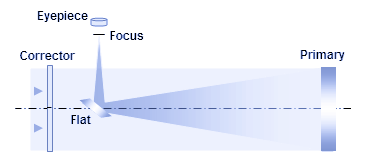telescopeѲptics.net          ▪▪▪▪                                             CONTENTS

# 10.2.2.3. Schmidt-Newton telescope

The only difference in the optical layout of the Schmidt-Newtonian vs. Schmidt camera is in the position of corrector lens. In the telescope, the corrector is typically positioned at a distance somewhat inside the focus of the spherical primary (FIG. 173). The reduced mirror-to-corrector distance has practically no effect on theFIGURE 173: Schmidt-Newtonian telescope optical elements. Schmidt corrector cancels spherical aberration of the spherical primary. It also usually supports the diagonal mount, which eliminates spider diffraction effect. Due to displaced stop (normally coinciding with the corrector), coma, astigmatism and field curvature are all lower than in the comparable Newtonian with paraboloidal primary with the stop at the surface (while the benefit of lower astigmatism or field curvature can be achieved by manipulating stop position of a paraboloid as well, its coma error is independent of stop position). While the corrector is very forgiving to miscollimation, it does complicate adjusting the flat.

needed corrector power to cancel spherical aberration of the mirror. Needed corrector shape is determined from Eq. 101 or Eq. 101.1 (higher-order aberration is negligible). However, as a consequence of the stop now being closer to the primary, portions of mirror's coma and astigmatism are reintroduced, which is evident on the ray spot plot. The P-V wavefront error of lower-order coma in the Schmidt-Newtonian for object at infinity is given by:

Wc = (1-σ)αD/48F2           (110)

with σ being the corrector-to-primary separation in units of the primary radius of curvature (σ is numerically positive), α the field angle in radians, D the aperture diameter and F the focal ratio. With α=h/ƒ, h being the linear height in the image plane, and ƒ the mirror focal length, it can be also expressed as Wc=(1-σ)h/48F3.

It makes coma in the Schmidt-Newtonian lower by a factor of (1-σ) vs. paraboloidal Newtonian.

The lower-order astigmatism P-V wavefront error is given by:

Wa  = (1-σ)2Dα2/8F          (111)

or, alternatively, Wa=-(1-σ)2h2/8DF3. In other words, it is by a factor (1-σ)2 lower than for a mirror with the stop at the surface. Change in astigmatism also changes the median (best) image surface. It is now given by:with the sagittal surface curvature radius 1/Rs=(2/R)-[2(1-σ)2/R], and the tangential surface curvature given by
1/R
t=(2/R)-[6(1-σ)2/R], R being the mirror radius of curvature. It makes median field curvature lower by a factor of [1-2(1-σ)2] than for a paraboloid with the stop at the surface.

With the usual value for σ of ~0.45, the Schmidt-Newtonian coma is lower by a factor of ~0.55, astigmatism by a factor of ~0.3, and median field curvature by a factor of ~0.4 vs. comparable paraboloid with the stop at the surface. Of course, similar gains in the reduction of astigmatism and field curvature can be just as well obtained with a paraboloid, by moving the stop away from the surface.

Elements alignment in the Schmidt-Newtonian is somewhat more complicated than in the all-reflecting arrangement. The two mirrors and the focuser have to be aligned as in all-reflecting system, but all three also need to be aligned with the corrector. Decentered corrector will induce coma, as given by Eq. 109, while corrector tilt will induce tilted image surface. With the corrector commonly supporting diagonal mount, the limit to collimation accuracy is set by the accuracy of the corrector/diagonal alignment (unless correction is made at the focuser).

On the other hand, better coma correction of Schmidt-Newtonian makes the tolerance for mirrors/focuser misalignment nearly twice more forgiving than in a comparable all-reflecting Newtonian.

10.2.2.2. Wright, Baker camera, Hyperstar   ▐    10.2.2.4. Schmidt-Cassegrain telescope